# 3 april '13 (everyone) on Singapore Maths for ICBB / PSLE Math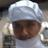English Teacher at Aoxin International School um Aoxin International School
11. Dec 2013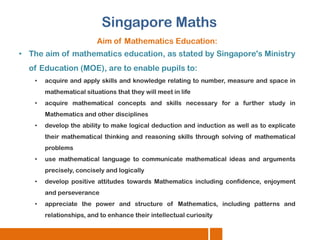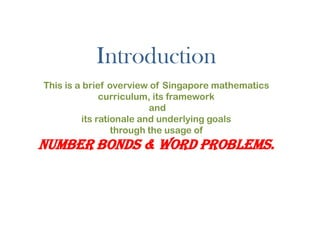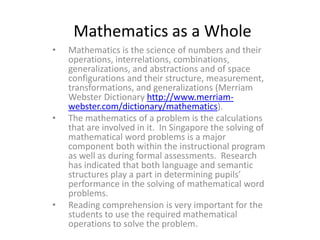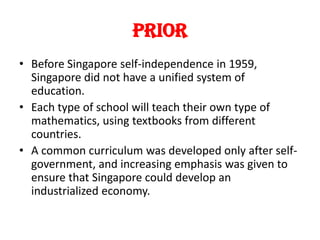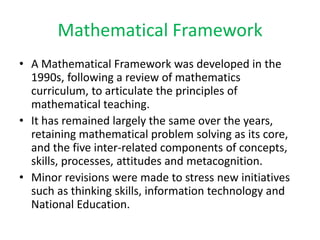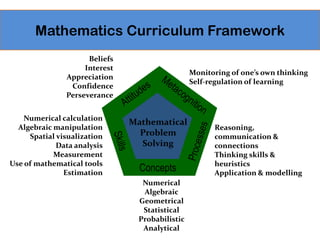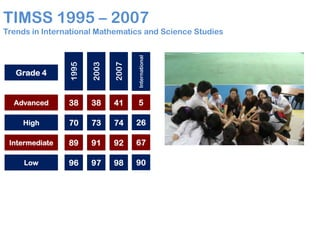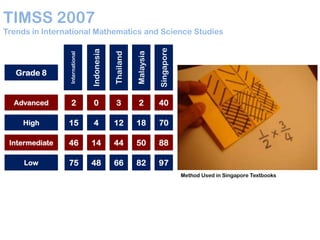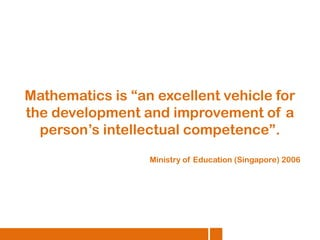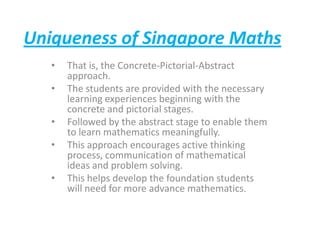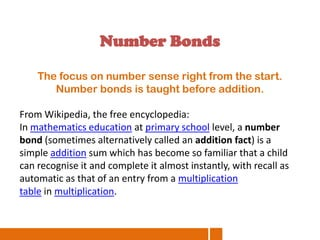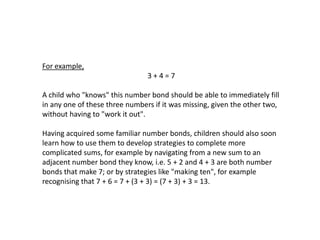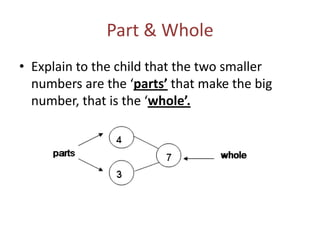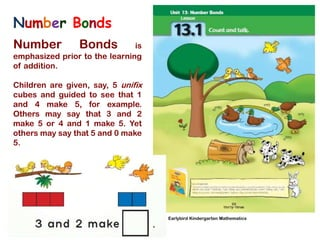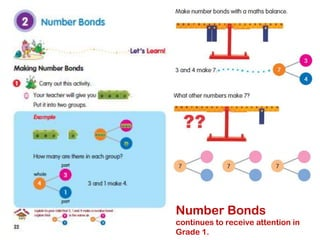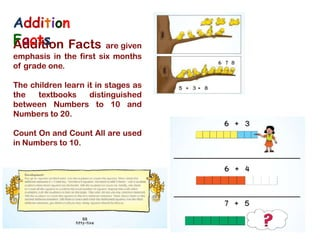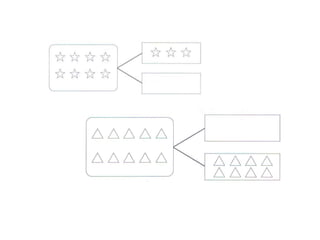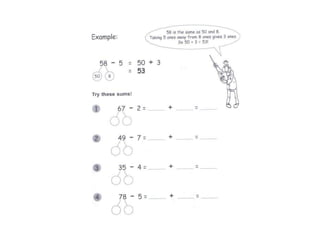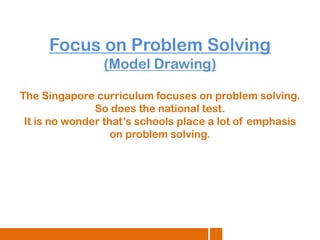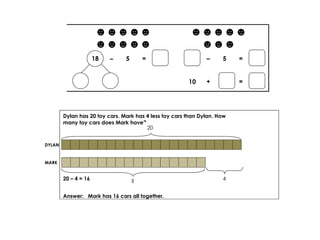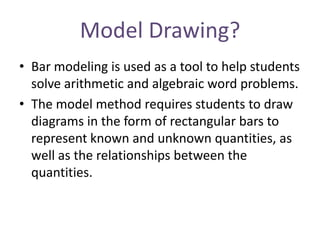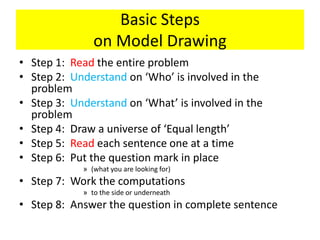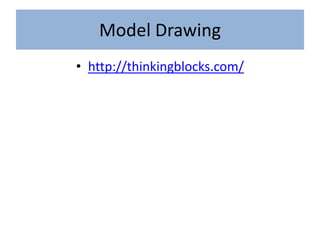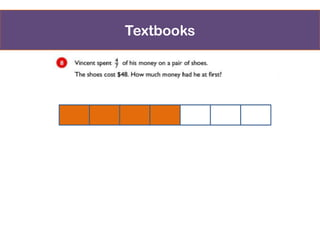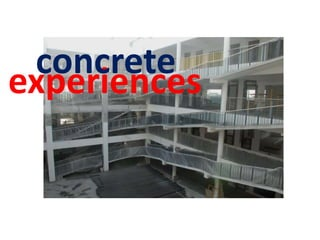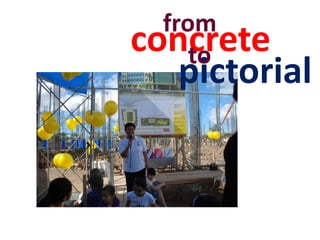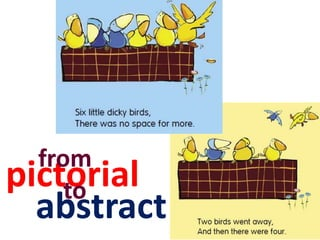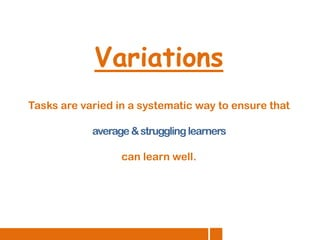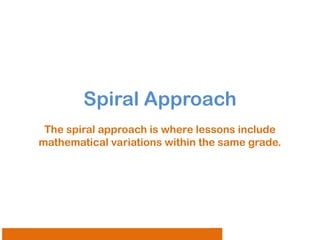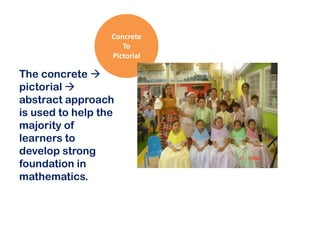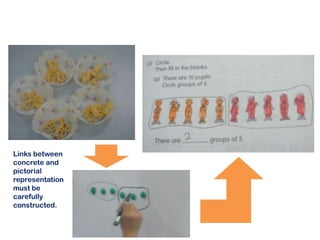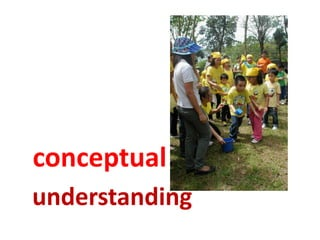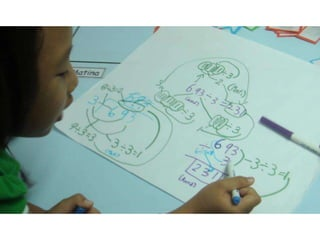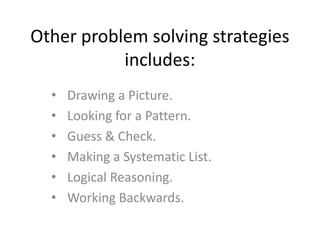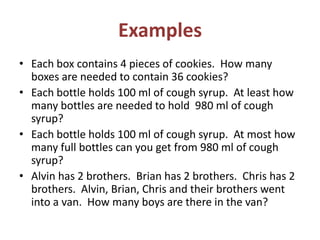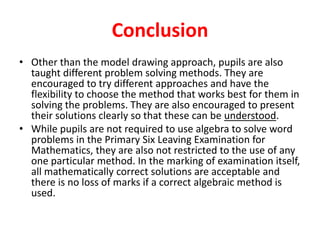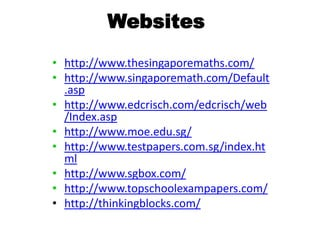1 von 37

### 3 april '13 (everyone) on Singapore Maths for ICBB / PSLE Math

• 1. Singapore Maths Aim of Mathematics Education: • The aim of mathematics education, as stated by Singapore's Ministry of Education (MOE), are to enable pupils to: • acquire and apply skills and knowledge relating to number, measure and space in mathematical situations that they will meet in life • acquire mathematical concepts and skills necessary for a further study in Mathematics and other disciplines • develop the ability to make logical deduction and induction as well as to explicate their mathematical thinking and reasoning skills through solving of mathematical problems • use mathematical language to communicate mathematical ideas and arguments precisely, concisely and logically • develop positive attitudes towards Mathematics including confidence, enjoyment and perseverance • appreciate the power and structure of Mathematics, including patterns and relationships, and to enhance their intellectual curiosity
• 2. Introduction This is a brief overview of Singapore mathematics curriculum, its framework and its rationale and underlying goals through the usage of Number Bonds & Word Problems.
• 3. Mathematics as a Whole • • • Mathematics is the science of numbers and their operations, interrelations, combinations, generalizations, and abstractions and of space configurations and their structure, measurement, transformations, and generalizations (Merriam Webster Dictionary http://www.merriamwebster.com/dictionary/mathematics). The mathematics of a problem is the calculations that are involved in it. In Singapore the solving of mathematical word problems is a major component both within the instructional program as well as during formal assessments. Research has indicated that both language and semantic structures play a part in determining pupils’ performance in the solving of mathematical word problems. Reading comprehension is very important for the students to use the required mathematical operations to solve the problem.
• 4. Prior • Before Singapore self-independence in 1959, Singapore did not have a unified system of education. • Each type of school will teach their own type of mathematics, using textbooks from different countries. • A common curriculum was developed only after selfgovernment, and increasing emphasis was given to ensure that Singapore could develop an industrialized economy.
• 5. Mathematical Framework • A Mathematical Framework was developed in the 1990s, following a review of mathematics curriculum, to articulate the principles of mathematical teaching. • It has remained largely the same over the years, retaining mathematical problem solving as its core, and the five inter-related components of concepts, skills, processes, attitudes and metacognition. • Minor revisions were made to stress new initiatives such as thinking skills, information technology and National Education.
• 6. Mathematics Curriculum Framework Beliefs Interest Appreciation Confidence Perseverance Numerical calculation Algebraic manipulation Spatial visualization Data analysis Measurement Use of mathematical tools Estimation Monitoring of one’s own thinking Self-regulation of learning Mathematical Problem Solving Concepts Numerical Algebraic Geometrical Statistical Probabilistic Analytical Reasoning, communication & connections Thinking skills & heuristics Application & modelling
• 7. TIMSS 1995 – 2007 Grade 4 1995 2003 2007 International Trends in International Mathematics and Science Studies Advanced 38 38 41 5 High 70 73 74 26 Intermediate 89 91 92 67 Low 96 97 98 90
• 8. TIMSS 2007 International Indonesia Thailand Malaysia Singapore Trends in International Mathematics and Science Studies Advanced 2 0 3 2 40 High 15 4 12 18 70 Intermediate 46 14 44 50 88 Low 75 48 66 82 97 Grade 8 Method Used in Singapore Textbooks
• 9. Mathematics is “an excellent vehicle for the development and improvement of a person’s intellectual competence”. Ministry of Education (Singapore) 2006
• 10. Uniqueness of Singapore Maths • • • • • That is, the Concrete-Pictorial-Abstract approach. The students are provided with the necessary learning experiences beginning with the concrete and pictorial stages. Followed by the abstract stage to enable them to learn mathematics meaningfully. This approach encourages active thinking process, communication of mathematical ideas and problem solving. This helps develop the foundation students will need for more advance mathematics.
• 11. Number Bonds The focus on number sense right from the start. Number bonds is taught before addition. From Wikipedia, the free encyclopedia: In mathematics education at primary school level, a number bond (sometimes alternatively called an addition fact) is a simple addition sum which has become so familiar that a child can recognise it and complete it almost instantly, with recall as automatic as that of an entry from a multiplication table in multiplication.
• 12. For example, 3+4=7 A child who "knows" this number bond should be able to immediately fill in any one of these three numbers if it was missing, given the other two, without having to "work it out". Having acquired some familiar number bonds, children should also soon learn how to use them to develop strategies to complete more complicated sums, for example by navigating from a new sum to an adjacent number bond they know, i.e. 5 + 2 and 4 + 3 are both number bonds that make 7; or by strategies like "making ten", for example recognising that 7 + 6 = 7 + (3 + 3) = (7 + 3) + 3 = 13.
• 13. Part & Whole • Explain to the child that the two smaller numbers are the ‘parts’ that make the big number, that is the ‘whole’.
• 14. Number Bonds Number Bonds is emphasized prior to the learning of addition. Children are given, say, 5 unifix cubes and guided to see that 1 and 4 make 5, for example. Others may say that 3 and 2 make 5 or 4 and 1 make 5. Yet others may say that 5 and 0 make 5. Earlybird Kindergarten Mathematics
• 16. Addition Facts Facts Addition are given emphasis in the first six months of grade one. The children learn it in stages as the textbooks distinguished between Numbers to 10 and Numbers to 20. Count On and Count All are used in Numbers to 10.
• 19. Focus on Problem Solving (Model Drawing) The Singapore curriculum focuses on problem solving. So does the national test. It is no wonder that’s schools place a lot of emphasis on problem solving.
• 20. ☻☻☻☻☻ ☻☻☻☻☻ 18 – 5 ☻☻☻☻☻ ☻☻☻ = – 10 5 + = Dylan has 20 toy cars. Mark has 4 less toy cars than Dylan. How many toy cars does Mark have? 20 DYLAN MARK 20 – 4 = 16 ? Answer: Mark has 16 cars all together. = 4
• 21. Model Drawing? • Bar modeling is used as a tool to help students solve arithmetic and algebraic word problems. • The model method requires students to draw diagrams in the form of rectangular bars to represent known and unknown quantities, as well as the relationships between the quantities.
• 22. Basic Steps on Model Drawing • Step 1: Read the entire problem • Step 2: Understand on ‘Who’ is involved in the problem • Step 3: Understand on ‘What’ is involved in the problem • Step 4: Draw a universe of ‘Equal length’ • Step 5: Read each sentence one at a time • Step 6: Put the question mark in place » (what you are looking for) • Step 7: Work the computations » to the side or underneath • Step 8: Answer the question in complete sentence
• 23. Model Drawing • http://thinkingblocks.com/
• 24. Textbooks
• 25. concrete experiences
• 26. from concrete to pictorial
• 27. from pictorial to abstract
• 28. Variations Tasks are varied in a systematic way to ensure that average & struggling learners can learn well.
• 29. Spiral Approach The spiral approach is where lessons include mathematical variations within the same grade.
• 30. Concrete To Pictorial The concrete  pictorial  abstract approach is used to help the majority of learners to develop strong foundation in mathematics.
• 31. Links between concrete and pictorial representation must be carefully constructed.
• 32. conceptual understanding
• 34. Other problem solving strategies includes: • • • • • • Drawing a Picture. Looking for a Pattern. Guess & Check. Making a Systematic List. Logical Reasoning. Working Backwards.
• 35. Examples • Each box contains 4 pieces of cookies. How many boxes are needed to contain 36 cookies? • Each bottle holds 100 ml of cough syrup. At least how many bottles are needed to hold 980 ml of cough syrup? • Each bottle holds 100 ml of cough syrup. At most how many full bottles can you get from 980 ml of cough syrup? • Alvin has 2 brothers. Brian has 2 brothers. Chris has 2 brothers. Alvin, Brian, Chris and their brothers went into a van. How many boys are there in the van?
• 36. Conclusion • Other than the model drawing approach, pupils are also taught different problem solving methods. They are encouraged to try different approaches and have the flexibility to choose the method that works best for them in solving the problems. They are also encouraged to present their solutions clearly so that these can be understood. • While pupils are not required to use algebra to solve word problems in the Primary Six Leaving Examination for Mathematics, they are also not restricted to the use of any one particular method. In the marking of examination itself, all mathematically correct solutions are acceptable and there is no loss of marks if a correct algebraic method is used.
• 37. Websites • http://www.thesingaporemaths.com/ • http://www.singaporemath.com/Default .asp • http://www.edcrisch.com/edcrisch/web /Index.asp • http://www.moe.edu.sg/ • http://www.testpapers.com.sg/index.ht ml • http://www.sgbox.com/ • http://www.topschoolexampapers.com/ • http://thinkingblocks.com/